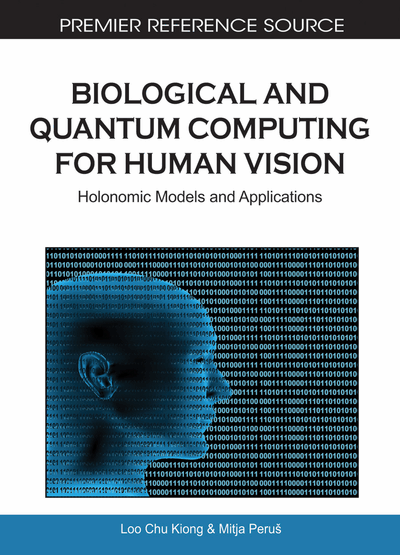# Images, Associations and Conscious Experience

DOI: 10.4018/978-1-61520-785-5.ch004
OnDemand:
(Individual Chapters)
Available
\$37.50
No Current Special Offers

Top

## 4.1 Convolution, Correlation, And Matrix-Processing

Pribram (1991, Lecture 10) presents indications that the far-frontal cortex prefers continuous processing and thus convolution / correlation processing. On the other hand, the posterior cerebral convexity prefers discrete processing and thus matrix (interference) processing. The model of section 1.2 is convolutional, as is evident from equation (1.1). My quantum associative net of section 1.4 and all the related models presented in detail in Auxil. Chapters (HNeT, the Hopfield and Haken ANN models) have a matrix-core, as in equation (1.4) where the elements of matrix G (similar to J) are given, but they take part in a sort of convolutional process – as in equation (1.5). Convolutional and matrix operations are thus often combined, and both can involve correlation-processing.

Convolution / correlation processing is more appropriate for configural perception, while matrix (interference) processing is more convenient for modeling associative cognitive processes like formation of prototypes.

Inner versus outer product. The key-difference is that convolutional computing incorporates the scalar or inner products of pattern-vectors, and matrix-computing uses a Hebb-based tensor or outer product of pattern-vectors. Computing scalar (inner) products gives an “enfolded” code — the result is a single number, i.e. an activity-parameter or probability-coefficient. Computing tensor (outer) products gives a distributed, “unfolded” encoding — the result is a whole matrix, i.e. an array of numbers–weights (which is like description of a hologram).1

The convolutional processing, as executed along the retino-geniculo-striate visual pathway2, is more economic and up-to-date than the matrix-processing is. Namely, a single coefficient, i.e. the scalar product which describes global correlation of images, is a much more economic code than the matrix of many elements where each of them describes a local correlation of image-patches (and the matrix as a whole represent the combined, overall correlation). Economic refers to a lower amount of used processing elements, and this brings abstraction and condensation of information. Up-to-date refers to serial convolving of the current input with the memory (encoded in weights which are often matrix-elements).

In a brief summary: convolutions are used in early abstraction and perception (which is configural and serial); tensor-product matrix-computations are used for description of associative memory and top-down- or memory-based operations including cognitive associations and categorization.3

The holonomic theory views imaging as based on convolving the various stages of primary visual processing. In contrast, “[…] Object perception […] depends on correlations among patterns in which centers of symmetry are determined by operations performed in the superior colliculus and the visuomotor system.” (Pribram, 1991, p. 261)

Top

## 4.2 Attempt Of An Integrated Model Of Image Processing In V1, And Beyond

Synopsis. The holonomic theory of the retino-geniculo-striate image processing was presented in section 1.2. It uses Gabor wavelets, eq. (1.2.), as “weighting”- or “filtering”-functions while performing convolution, eq. (1.1), with the retinal image. The result of this Gabor transform is a spectral image-representation in V1. This, roughly hologram-like, representation in V1 is then reconstructed by an inverse Gabor-transform into the spatial representation in V2, probably. Thus, the topologically-correct “image” is recovered in inverted form in V2.4

## Complete Chapter List

Search this Book:
Reset